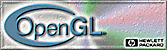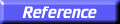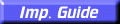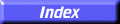# gluPartialDisk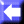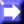gluPartialDisk: draw an arc of a disk.

##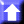C Specification

```void gluPartialDisk(
GLdouble	 inner,
GLdouble	 outer,
GLint	 slices,
GLint	 loops,
GLdouble	 start,
GLdouble	 sweep)
```

##Parameters

inner
Specifies the inner radius of the partial disk (can be 0).
outer
Specifies the outer radius of the partial disk.
slices
Specifies the number of subdivisions around the Z axis.
loops
Specifies the number of concentric rings about the origin into which the partial disk is subdivided.
start
Specifies the starting angle, in degrees, of the disk portion.
sweep
Specifies the sweep angle, in degrees, of the disk portion.

##Description

gluPartialDisk renders a partial disk on the Z = 0 plane. A partial disk is similar to a full disk, except that only the subset of the disk from start through start + sweep is included (where 0 degrees is along the +Y axis, 90 degrees along the +X axis, 180 along the
Y axis, and 270 along theX axis).

The partial disk has a radius of outer, and contains a concentric circular hole with a radius of inner. If inner is 0, then no hole is generated. The partial disk is subdivided around the Z axis into slices (like pizza slices), and also about the Z axis into rings (as specified by slices and loops, respectively).

With respect to orientation, the +Z side of the partial disk is considered to be outside (see gluQuadricOrientation). This means that if the orientation is set to GLU_OUTSIDE, then any normals generated point along the +Z axis. Otherwise, they point along theZ axis.

If texturing is turned on (with gluQuadricTexture), texture coordinates are generated linearly such that where r = outer, the value at (r, 0, 0) is (1.0, 0.5), at (0, r, 0) it is (0.5, 1.0), at (r, 0, 0) it is (0.0, 0.5), and at (0,r, 0) it is (0.5, 0.0).

gluCylinder,
gluDisk,